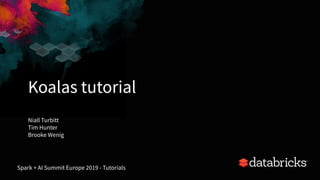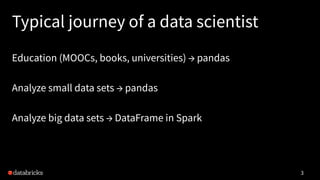Successfully reported this slideshow.

# Koalas: Pandas on Apache Spark×

# Koalas: Pandas on Apache Spark

In this tutorial we will present Koalas, a new open source project that we announced at the Spark + AI Summit in April. Koalas is an open-source Python package that implements the pandas API on top of Apache Spark, to make the pandas API scalable to big data. Using Koalas, data scientists can make the transition from a single machine to a distributed environment without needing to learn a new framework.

We will demonstrate Koalas’ new functionalities since its initial release, discuss its roadmaps, and how we think Koalas could become the standard API for large scale data science.

What you will learn:

How to get started with Koalas
Easy transition from Pandas to Koalas on Apache Spark
Similarities between Pandas and Koalas APIs for DataFrame transformation and feature engineering
Single machine Pandas vs distributed environment of Koalas
Prerequisites:

A fully-charged laptop (8-16GB memory) with Chrome or Firefox
Python 3 and pip pre-installed
pip install koalas from PyPI
Pre-register for Databricks Community Edition

In this tutorial we will present Koalas, a new open source project that we announced at the Spark + AI Summit in April. Koalas is an open-source Python package that implements the pandas API on top of Apache Spark, to make the pandas API scalable to big data. Using Koalas, data scientists can make the transition from a single machine to a distributed environment without needing to learn a new framework.

We will demonstrate Koalas’ new functionalities since its initial release, discuss its roadmaps, and how we think Koalas could become the standard API for large scale data science.

What you will learn:

How to get started with Koalas
Easy transition from Pandas to Koalas on Apache Spark
Similarities between Pandas and Koalas APIs for DataFrame transformation and feature engineering
Single machine Pandas vs distributed environment of Koalas
Prerequisites:

A fully-charged laptop (8-16GB memory) with Chrome or Firefox
Python 3 and pip pre-installed
pip install koalas from PyPI
Pre-register for Databricks Community Edition

### Koalas: Pandas on Apache Spark

1. 1. Koalas tutorial 1 Spark + AI Summit Europe 2019 - Tutorials Niall Turbitt Tim Hunter Brooke Wenig
2. 2. About Niall Turbitt, Data Scientist at Databricks • Professional services and training • MSc Statistics University College Dublin, B.A Mathematics & Economics Trinity College Dublin Tim Hunter, Software Engineer at Databricks • Co-creator of the Koalas project • Contributes to Apache Spark MLlib, GraphFrames, TensorFrames and Deep Learning Pipelines libraries • Ph.D Machine Learning from Berkeley, M.S. Electrical Engineering from Stanford
3. 3. Typical journey of a data scientist Education (MOOCs, books, universities) → pandas Analyze small data sets → pandas Analyze big data sets → DataFrame in Spark 3
4. 4. pandas Authored by Wes McKinney in 2008 The standard tool for data manipulation and analysis in Python Deeply integrated into Python data science ecosystem, e.g. numpy, matplotlib Can deal with a lot of diﬀerent situations, including: - basic statistical analysis - handling missing data - time series, categorical variables, strings 4
5. 5. Apache Spark De facto unified analytics engine for large-scale data processing (Streaming, ETL, ML) Originally created at UC Berkeley by Databricks’ founders PySpark API for Python; also API support for Scala, R and SQL 5
6. 6. Koalas Announced April 24, 2019 Pure Python library Aims at providing the pandas API on top of Apache Spark: - unifies the two ecosystems with a familiar API - seamless transition between small and large data 6
7. 7. Quickly gaining traction 7 Bi-weekly releases! > 500 patches merged since announcement > 20 significant contributors outside of Databricks > 8k daily downloads
8. 8. 8 pandas DataFrame Spark DataFrame Column df['col'] df['col'] Mutability Mutable Immutable Add a column df['c'] = df['a'] + df['b'] df.withColumn('c', df['a'] + df['b']) Rename columns df.columns = ['a','b'] df.select(df['c1'].alias('a'), df['c2'].alias('b')) Value count df['col'].value_counts() df.groupBy(df['col']).count().order By('count', ascending = False) pandas DataFrame vs Spark DataFrame
9. 9. A short example 9 import pandas as pd df = pd.read_csv("my_data.csv") df.columns = ['x', 'y', 'z1'] df['x2'] = df.x * df.x df = (spark.read .option("inferSchema", "true") .option("comment", True) .csv("my_data.csv")) df = df.toDF('x', 'y', 'z1') df = df.withColumn('x2', df.x*df.x)
10. 10. A short example 10 import pandas as pd df = pd.read_csv("my_data.csv") df.columns = ['x', 'y', 'z1'] df['x2'] = df.x * df.x import databricks.koalas as ks df = ks.read_csv("my_data.csv") df.columns = ['x', 'y', 'z1'] df['x2'] = df.x * df.x
11. 11. Current status Bi-weekly releases, very active community with daily changes The most common functions have been implemented: - 60% of the DataFrame / Series API - 60% of the DataFrameGroupBy / SeriesGroupBy API - 15% of the Index / MultiIndex API - to_datetime, get_dummies, … 11
12. 12. New features - 80% of the plot functions (0.16.0-) - Spark related functions (0.8.0-) - IO: to_parquet/read_parquet, to_csv/read_csv, to_json/read_json, to_spark_io/read_spark_io, to_delta/read_delta, ... - SQL - cache - Support for multi-index columns (90%) (0.16.0-) - Options to configure Koalas’ behavior (0.17.0-) 12
13. 13. Challenge: increasing scale and complexity of data operations Struggling with the “Spark switch” from pandas More than 10X faster with less than 1% code changes How Virgin Hyperloop One reduced processing time from hours to minutes with Koalas
14. 14. Key Diﬀerences Spark is more lazy by nature: - most operations only happen when displaying or writing a DataFrame Spark does not maintain row order Performance when working at scale 14
15. 15. InternalFrame 15 Koalas DataFrame InternalFrame - column_index - index_map Spark DataFrame
16. 16. InternalFrame 16 Koalas DataFrame InternalFrame - column_index - index_map Spark DataFrame InternalFrame - column_index - index_map Spark DataFrame Koalas DataFrame API call copy with new state
17. 17. Notebooks 17 bit.ly/koalas_1_sseu bit.ly/koalas_2_sseu
18. 18. Thank you! github.com/databricks/koalas Get Started at databricks.com/try 18
19. 19. Internal Frame Internal immutable metadata. - holds the current Spark DataFrame - manages mapping from Koalas column names to Spark column names - manages mapping from Koalas index names to Spark column names - converts between Spark DataFrame and pandas DataFrame 19
20. 20. InternalFrame 20 Koalas DataFrame InternalFrame - column_index - index_map Spark DataFrame InternalFrame - column_index - index_map Koalas DataFrame API call copy with new state Only updates metadata kdf.set_index(...)
21. 21. InternalFrame 21 Koalas DataFrame InternalFrame - column_index - index_map Spark DataFrame InternalFrame - column_index - index_map Spark DataFrame API call copy with new state e.g., inplace=True kdf.dropna(..., inplace=True)
22. 22. Operations on diﬀerent DataFrames We only allow Series derived from the same DataFrame by default. 22 OK - df.a + df.b - df['c'] = df.a * df.b Not OK - df1.a + df2.b - df1['c'] = df2.a * df2.b
23. 23. Operations on diﬀerent DataFrames We only allow Series derived from the same DataFrame by default. Equivalent Spark code? 23 OK - df.a + df.b - df['c'] = df.a * df.b sdf.select( sdf['a'] + sdf['b']) Not OK - df1.a + df2.b - df1['c'] = df2.a * df2.b ??? sdf1.select( sdf1['a'] + sdf2['b'])
24. 24. Operations on diﬀerent DataFrames We only allow Series derived from the same DataFrame by default. Equivalent Spark code? 24 OK - df.a + df.b - df['c'] = df.a * df.b sdf.select( sdf['a'] + sdf['b']) Not OK - df1.a + df2.b - df1['c'] = df2.a * df2.b ??? sdf1.select( sdf1['a'] + sdf2['b'])AnalysisException!!
25. 25. Operations on diﬀerent DataFrames ks.set_option('compute.ops_on_diff_frames', True) Equivalent Spark code? 25 OK - df.a + df.b - df['c'] = df.a * df.b sdf.select( sdf['a'] + sdf['b']) OK - df1.a + df2.b - df1['c'] = df2.a * df2.b sdf1.join(sdf2, on="_index_") .select('a * b')
26. 26. Default Index Koalas manages a group of columns as index. The index behaves the same as pandas’. If no index is specified when creating a Koalas DataFrame: it attaches a “default index” automatically. Each “default index” has Pros and Cons. 26
27. 27. Default Indexes Configurable by the option “compute.default_index_type” See also: https://koalas.readthedocs.io/en/latest/user_guide/options.html#default-index-type 27 requires to collect data into single node requires shuffle continuous increments sequence YES YES YES distributed-sequence NO YES / NO YES / NO distributed NO NO NO
28. 28. What to expect? • Improve pandas API coverage - rolling/expanding • Support categorical data types • More time-series related functions • Improve performance - Minimize the overhead at Koalas layer - Optimal implementation of APIs 28
29. 29. Getting started pip install koalas conda install koalas Look for docs on https://koalas.readthedocs.io/en/latest/ and updates on github.com/databricks/koalas 10 min tutorial in a Live Jupyter notebook is available from the docs. 29
30. 30. Do you have suggestions or requests? Submit requests to github.com/databricks/koalas/issues Very easy to contribute koalas.readthedocs.io/en/latest/development/contributing.html 30
31. 31. Koalas Sessions Koalas: Pandas on Apache Spark (Tutorial) - 14:30 - @ROOM: G104 AMA: Koalas - 16:00 - @EXPO HALL 31
32. 32. Apache Spark: I unify data engineering and data science! Data Scientist: I like pandas! Apache Spark: pandas does not scale! Data Scientist: I still like pandas! Apache Spark: pandas lacks parallelism! Data Scientist: I still like pandas! Apache Spark: OK… How about Koalas? Data Scientist: I like Koalas! Awesome!
33. 33. Why do we need Koalas? 1. Data scientists write a bunch of models and codes in pandas 2. Data scientists are told to make it distributed at some point because the business got bigger. 3. Struggling and frustrated at all other popular distributed systems. 4. Ask some help to some engineers 5. Engineers have no idea what models the data scientists wrote in pandas are. Data scientists have no idea why the engineers can’t make it working. 6. They fight with each other and get fed up with that. 7. End up with something lousy and just-work-for-now. 8. Thousands of bugs are found. Both engineers and data scientists don’t know what’s going on and keep tossing those bugs to each other. 9. Go to 10. or they quit their job
34. 34. import pandas as pd df = pd.read_csv('my_data.csv') df.columns = ['x', 'y', 'z1'] df['x2'] = df.x * df.x import databricks.koalas as ks df = ks.read_csv('my_data.csv') df.columns = ['x', 'y', 'z1'] df['x2'] = df.x * df.x Instead of pandas … … simply say Koalas